### Day 45 - Quadratics Practice - 10.21.14

 UpdatesNew Seats!Quadratic Test on Friday, 10/24!Bell RingerWhat are the roots (solutions) of the graph of the quadratic equation?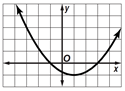–1 and 3–3 and 1–1 and 11 and 3Find the coordinates of the vertex of the graph of y = 4-x2.  Identify the vertex as a maximum or a minimum.(2, 0); maximum(0, 4); maximum(0, 4); minimum(2, 0); minimumWhich equation corresponds to the graph?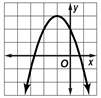y = x2 - 2x + 2y = -x2 + 2x + 2y = x2 + 2x + 2y = -x2 - 2x + 2Which graph corresponds with the equation y = (x - 2)2 - 2?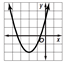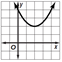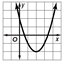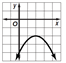Which quadratic function is equivalent to y = x2 + 2x - 3?y = (x + 1)2 - 2y = (x - 1)2 - 4y = (x - 1)2 - 2y = (x + 1)2 - 4ReviewPrerequisitesDegree of a PolynomialZero Product PropertyQuadratic FunctionsParabolaMinimum/MaximumFinding by using algebra or graphingVertexFinding by using algebra or graphingAxis of Symmetryx = -b / (2a)Quadratic Roots (x-intercepts)Vertex FormFactored FormCompleting the SquareExample problemsQuadratic FormulaLessonComplex SolutionsProgrammingAxis of SymmetryVertexComplex SolutionsBrainstorming ActivityDescribe the difference between a parabola with a maximum and a parabola with a minimum value.Give examples of two different parabolas that have the same vertex.Explain how the axis of symmetry can help graph a quadratic function.Exit TicketPosted on board at end of block. Lesson Objective(s)How can the quadratic formula be used to solve problems?Standard(s)Graphing Quadratic FunctionsCC.9-12.F.IF.7a Graph linear and quadratic functions and show intercepts, maxima, and minima.Solving Quadratic EquationsCC.9-12.A.REI.4 Solve equations and inequalities in one variable. Solve quadratic equations in one variable.CC.9-12.A.REI.4b Solve quadratic equations by inspection (e.g., for x^2 = 49), taking square roots, completing the square, the quadratic formula and factoring, as appropriate to the initial form of the equation. Recognize when the quadratic formula gives complex solutions and write them as a ± bi for real numbers a and b.Factored FormCC.9-12.A.SSE.3a Factor a quadratic expression to reveal the zeros of the function it defines.CC.9-12.F.IF.8a Use the process of factoring and completing the square in a quadratic function to show zeros, extreme values, and symmetry of the graph, and interpret these in terms of a context.Completing the SquareCC.9-12.A.SSE.3b Complete the square in a quadratic expression to reveal the maximum or minimum value of the function it defines.CC.9-12.A.REI.4a Use the method of completing the square to transform any quadratic equation in x into an equation of the form (x – p)^2 = q that has the same solutions. Derive the quadratic formula from this form.CC.9-12.F.IF.8a Use the process of factoring and completing the square in a quadratic function to show zeros, extreme values, and symmetry of the graph, and interpret these in terms of a context.Quadratic FormulaCC.9-12.A.REI.4a Use the method of completing the square to transform any quadratic equation in x into an equation of the form (x – p)^2 = q that has the same solutions. Derive the quadratic formula from this form.Creating Quadratic EquationsCC.9-12.A.CED.1 Create equations that describe numbers or relationship. Create equations and inequalities in one variable and use them to solve problems. Include equations arising from linear and quadratic functions, and simple rational and exponential functions.Comparing Quadratics Function with Different RepresentationsCC.9-12.F.IF.9 Analyze functions using different representations. Compare properties of two functions each represented in a different way (algebraically, graphically, numerically in tables, or by verbal descriptions). For example, given a graph of one quadratic function and an algebraic expression for another, say which has the larger maximum.Quadratic ModelingCC.9-12.F.LE.1 Construct and compare linear, quadratic, and exponential models and solve problems. Distinguish between situations that can be modeled with linear functions and with exponential functions.CC.9-12.S.ID.6a Fit a function to the data; use functions fitted to data to solve problems in the context of the data. Use given functions or choose a function suggested by the context. Emphasize linear, quadratic, and exponential models.Complex Solutions of Quadratic FunctionsCC.9-12.N.CN.7 Use complex numbers in polynomial identities and equations. Solve quadratic equations with real coefficients that have complex solutions.CC.9-12.A.REI.4b Solve quadratic equations by inspection (e.g., for x^2 = 49), taking square roots, completing the square, the quadratic formula and factoring, as appropriate to the initial form of the equation. Recognize when the quadratic formula gives complex solutions and write them as a ± bi for real numbers a and b.Mathematical Practice(s)#1 - Make sense of problems and persevere in solving them#2 - Reason abstractly and quantitatively#4 - Model with mathematics#5 - Use appropriate tools strategically#7 - Look for and make use of structurePast CheckpointsGraphing Quadratic Functionspage 528-529A - #8B - #34C - #36D - #41-43Solving Quadratic Equations by Graphingpage 536-538E - #16F - #18G - #20H - #40I - #50Solving Quadratic Equations by Completing the Squarepage 542-543J - #4K - #6L - #12M - #14N - #44O - #50Practice ProblemsQuadratic Formulapage 550-551P - #8Q - #22R - #30S - #32T - #34U - #48V - #50